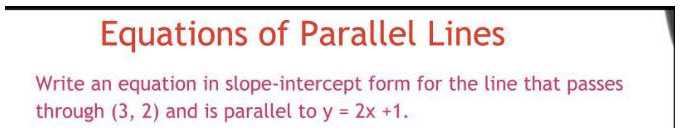### Still have math questions?Equations of Parallel Lines Write an equation in slope-intercept form for the line that passes through $$( 3,2 )$$ and is parallel to $$y = 2 x + 1$$ .
The equation of the line in the slope-intercept form is $$y = 2 x + 1$$ . The slope of the parallel line is the same: $$m = 2$$ . So, the equation of the parallel line is $$y = 2 x + a$$ . To find $$a$$ , we use the fact that the line should pass through the given point: $$2 = ( 2 ) \cdot ( 3 ) + a$$ . Thus, $$a = - 4$$ . Therefore, the equation of the line is $$y = 2 x - 4$$ .